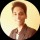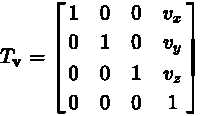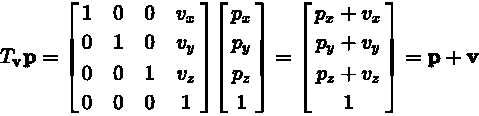Robotics and DIY Enthusiasts
Question, Answers and Discussions about DIY Robotics Projects for all tinkering lovers!
144 Members
Join this group to post and comment.Kunal Gokhe
Mechanical
25 Jan 2019

# Use of translation matrix in robotics

Matrix is very useful in robotics it's make complicated problems into simple mathematical problems. Translation matrix used in drones, robotic arms to move the end effector in linear manner. Translation matrix expression are given below.

To translate an object by vector v, each homogeneous vector p (written in homogeneous coordinates) can be multiplied by this translation matrix :The multiplication will give the expected result: